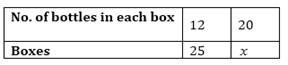# A batch of bottles was packed in 25 boxes with 12 bottles in each box. If the same batch is packed using 20 bottles in each box, how many boxes would be filled?

Solution

Let the number of boxes be x.the number of bottles and the number of boxes are inversely proportional.

Therefore, from inverse proportionality property:-

12/20 = x/25

12×25 = 20x

x = 12×25/20 = 15

Hence 15 boxes would be filled.(4)(0)